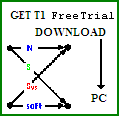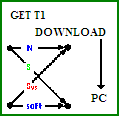Last Update: March 13, 2010, October 16, 2011.

First, consider the following Description of a MathWorks Product:

MATLABÛ (short for "matrix laboratory") is a programming system that performs a wide range of numerical computations. MATLABÛ has been designed to make use of highly efficient numerical methods for solving problems in applied mathematics, such as linear algebra, FFT, integration of functions, solving nonlinear equations, and solving ordinary differential equations. MATLABÛ has an extensive graphics capabilities for 2-D and 3-D including animation and color. Since MATLABÛ has its own programming language, it is highly extensible, allowing users to write their own applications, complete with a customized X-Windows-based user interface.

Next, AdvDCSMT1DCSS T1's purpose is not to provide low level numerical methods which is what MATLABÛ provides. T1's purpose is to model and simulate reliably Information Theoretic Systems to obtain a Performance Measurement of Reliability.

MATLABÛ is an ERECTER SET of numerical methods.

To do Coded or UnCoded Communications Systems Modeling and Simulation using MATLABÛ, a person (Non-student) needs to have:

1) MATLABÛ for \$2000;

2) Signal Processing Toolbox for \$1000;

3) Communications Toolbox™ for \$1000;

for a Total Cost (U.S. or Canada only) : \$4000 + Tax;

and, if needed, Training Classes for \$2300 (MATLABÛ Fundamentals & MATLABÛ for Building Graphical User Interfaces) and up (plus \$1,100 for Signal Processing with MATLABÛ plus \$650 for MATLABÛ Programming Techniques);

and a Huge amount of Time for each System design. If an error is made in a simulated system model, loss of valuable simulation time has occurred (could be a long time depending on the circumstances, days, weeks, months).

Note:

MathLabÛ and the required toolboxes prices are based on the MathWorks' 'The MathWorks Products and Prices North America Individual January 2010' pricing document that was published as of March 2010.

The student's version of MATLABÛ (& associated MathWorks' software) DOES NOT contain the Communications Toolbox. The Communications Toolbox is an Add-On product from Mathworks™.

The cost for this student's version of MATLABÛ (& associated MathWorks' software and Add-on Communications Toolbox) with a Student License is about \$129 (\$100 plus \$29) + Tax.

The student version of the MATLABÛ software requires proof of being a student and is strictly prohibited for Commercial use, including commercial research.

MATLABÛ's programming language is based on scripting (cryptic/abbreviated scripts or commands): it should be obvious why Training classes would be needed to use MATLABÛ efficiently.

Check out a comment on MATLABÛ by another Electrical Engineer!

NSSyssoft's System tool T1 (version 1.x) for \$55.00 addresses the Needs for a RELIABLE Computational Science and Engineering Systems Tool. MATLABÛ has none of these. This Advanced Digital Communication System Modeler (AdvDCSM) Technology section of this website discusses these Needs. The technology in T1 is first applied to Coded and UnCoded Digital Communications Systems. This work began in 1999 with the development of Technology (Expert System & Intelligent Agents) and the T1 Product (Communications System Modeler and Simulator).

T1 is NOW AVAILABLE as a TRIAL VERSION that will allow you to use T1, a Digital Communication (Information-Theoretic) System Modeling and Simulation software tool, for a Limited Period without Charge and to learn about the valuable utility of T1 in the study, evaluation, and possibly design of certain Complex Digital Communication Systems first hand before purchase.

```GET FREE TRIAL USE of T1, AdvDCSMT1DCSS by DOWNLOAD.
```
```BUY T1 (ADVDCSMT1DCSS software system tool)NOW.